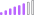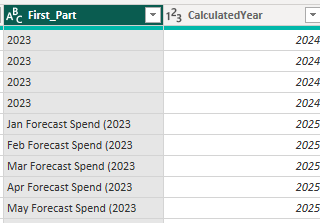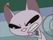cancel
Showing results for
Did you mean:Post Patron

## Replace a number in a string with another one

Hello,

I have a column that contains a number (a year), which is NOT FIXED - it could be any year.

I need to replace it with the values of another column:In this case, what in the column [First_Part] reads 2023 should be replaced with the corresponding CalculatedYear - and the additional text should be kept.

E.g., Jan Forecast Spend(2023 should be transformed into Jan Forecast Spend (2025

And again it should all be flexible - I cannot use 2023 as a reference... it's replacing the only number that will be in that column.

Thanks!

Kind regards

Valeria

1 ACCEPTED SOLUTIONSuper User

Hi Valeria,

Paste this into a new blank query using Advanced Editor to see the steps to take:

``````let
Source = Table.FromRows(Json.Document(Binary.Decompress(Binary.FromText("i45WMjIwMlbSAVEmSrE60UpeiXkKbs4KwQWpeSkKGuiyvolFCm7JSLImEFkLsKxLajKqrClY1thUKTYWAA==", BinaryEncoding.Base64), Compression.Deflate)), let _t = ((type nullable text) meta [Serialized.Text = true]) in type table [First_Part = _t, CalculatedYear = _t]),
chgTypes = Table.TransformColumnTypes(Source,{{"First_Part", type text}, {"CalculatedYear", Int64.Type}}),
splitColByDelim = Table.SplitColumn(chgTypes, "First_Part", Splitter.SplitTextByEachDelimiter({" ("}, QuoteStyle.Csv, true), {"First_Part.1", "First_Part.2"}),
addV2 = Table.AddColumn(splitColByDelim, "First_Part_v2", each if try Value.Is(Number.From([First_Part.1]), type number) otherwise false = true then Text.From([CalculatedYear])
else [First_Part.1] & " " & Text.From([CalculatedYear]))
in

I basically just split the original column by delimiter ( " (" ) then did a check to see if the original column value was numerical. If yes, then use [CalculatedYear], if no, then concatenate the split original with [CalculatedYear].

Example output:Pete

Now accepting Kudos! If my post helped you, why not give it a thumbs-up?

Proud to be a Datanaut!

8 REPLIES 8Memorable Member

Hi, @ValeriaBreve suppose that this is the only "number" in the string. Then

``````let
Source = Table.FromRows(Json.Document(Binary.Decompress(Binary.FromText("i45W8krMU3DLL0pNTiwuUTAyMDLWUNJRAtImSrE60SCGMZwfCwA=", BinaryEncoding.Base64), Compression.Deflate)), let _t = ((type nullable text) meta [Serialized.Text = true]) in type table [First_Part = _t, CalculatedYear = _t]),
numbers = List.Buffer({"0".."9"}),
r = Table.ToRecords(Source),
txf_field =
List.Transform(
r,
(x) =>
Record.TransformFields(
x,
{"First_Part", (y) => [s = Text.Select( y, numbers), u = Text.Replace(y, s, x[CalculatedYear])][u]}
)
),
result = Table.FromRecords(txf_field)
in
result``````Post Patron

@AlienSx Hi, this works as well! However, I have a hard time to follow the steps. I did learn the function Record. TransformFields that I did not know existed! But I am not good enough for now to follow through the function in function step. I will get there in time ;-).

Thanks!

Kind regards

ValeriaMemorable Member

``````[s = Text.Select( y, numbers), u = Text.Replace(y, s, x[CalculatedYear])][u]
// is just a replacement to
let
s = Text.Select( y, numbers),
u = Text.Replace(y, s, x[CalculatedYear])
in
u``````

You may think of a query as a record with step_names = record field names. You may use "let ... in" instead - nothing wrong with that.

Text.Select (y, numbers) selects only chars "0".."9" from your string. Result is a concatenation of all "numerical" chars in your string. Next step replaces this substring with a text value from your CalculatedYear column.

p.s. I've just noticed that CalculatedYear is Int64.Type. So please wrap x[CalculatedYear] in Text.From.Post Patron

@AlienSx thank you so much for taking the time to explain!!!!!! It is much clearer now. 🙂Super User

Hi Valeria,

Paste this into a new blank query using Advanced Editor to see the steps to take:

``````let
Source = Table.FromRows(Json.Document(Binary.Decompress(Binary.FromText("i45WMjIwMlbSAVEmSrE60UpeiXkKbs4KwQWpeSkKGuiyvolFCm7JSLImEFkLsKxLajKqrClY1thUKTYWAA==", BinaryEncoding.Base64), Compression.Deflate)), let _t = ((type nullable text) meta [Serialized.Text = true]) in type table [First_Part = _t, CalculatedYear = _t]),
chgTypes = Table.TransformColumnTypes(Source,{{"First_Part", type text}, {"CalculatedYear", Int64.Type}}),
splitColByDelim = Table.SplitColumn(chgTypes, "First_Part", Splitter.SplitTextByEachDelimiter({" ("}, QuoteStyle.Csv, true), {"First_Part.1", "First_Part.2"}),
addV2 = Table.AddColumn(splitColByDelim, "First_Part_v2", each if try Value.Is(Number.From([First_Part.1]), type number) otherwise false = true then Text.From([CalculatedYear])
else [First_Part.1] & " " & Text.From([CalculatedYear]))
in

I basically just split the original column by delimiter ( " (" ) then did a check to see if the original column value was numerical. If yes, then use [CalculatedYear], if no, then concatenate the split original with [CalculatedYear].

Example output:Pete

Now accepting Kudos! If my post helped you, why not give it a thumbs-up?

Proud to be a Datanaut!Post Patron

@BA_Pete Hi Pete, thanks! This works very well! I was trying to understand the code and there is something I cannot grasp.

When you write:

each try Value.Is(Number.From([First_Part.1]), type number) otherwise false = true

I do see it works, it converts the record from the "try" expression into the right "true/false" string without expanding any columns - but could you please elaborate into more details? I cannot figure it out.

Thanks!!!

Kind regards

ValeriaSuper User

Haha, yes, when you highlight it like that it does look a bit odd "otherwise false = true" 🙂

It actually breaks down like this:

Number.From( ... ) tries to convert your value to a number. It outputs an error if it's not.

Value.Is( ... , type number) tries to turn the result of the above into a TRUE/FALSE output to satisfy the IF...THEN structure. The problem with this is that if your value isn't a number, it evaluates to an error due to the error thrown by our Number.From step. So =>

try ... otherwise false handles this error by saying "TRY to get a non-error value from the above OTHERWISE give a FALSE output instead".

We now have an expression that always evaluates to either TRUE or FALSE with no errors, so we can use the IF...THEN...ELSE structure around it. So we end up with this:

if [the above evaluation] = true then [do this] else [do this]

Hope this makes sense.

Pete

Now accepting Kudos! If my post helped you, why not give it a thumbs-up?

Proud to be a Datanaut!Post Patron

@BA_Pete Hi Pete, got it, thanks!!!! 🙂

Very clear. So actually Powerquery reads it logically in the order of operations even without brackets...

I learned something today - THANKS!!! 🙂Announcements#### Exclusive opportunity for Women!

Join us for a free, hands-on Microsoft workshop led by women trainers for women where you will learn how to build a Dashboard in a Day!#### Power Platform Conference-Power BI and Fabric Sessions

Join us Oct 1 - 6 in Las Vegas for the Microsoft Power Platform Conference.Top Solution Authors
Top Kudoed Authors
Users online (2,481)